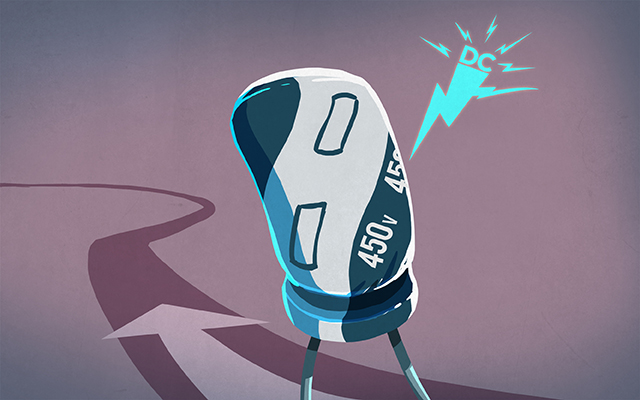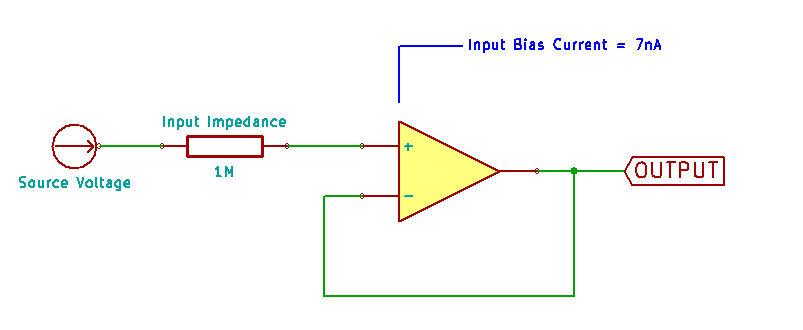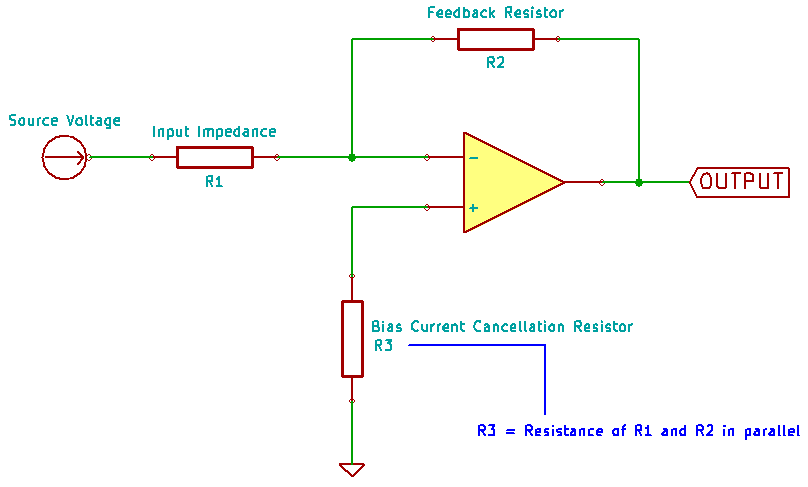# What’s all this “input bias current” anyhow?

An ideal op-amp has no current flow at the inputs. The world is not ideal.

In the real world, tiny amounts of current actually do flow into both the non-inverting and inverting inputs of your component. These currents are referred to as the input bias currents, IB+ and IB-. The causes of them are inherent to the design of the op-amp. They are used to bias the internal FETs or BJTs that make up the internal circuitry of the part. As with the input offset voltage, the non-inverting and the inverting bias current values may be very close, but not the same. The difference of the two values, (IB+) – (IB-), is the Input Offset Current.The bias currents are important, because they will create a voltage across any input impedance that might exist. Consider a very simple example: you have a DC signal source that is very, very high impedance, let’s say 1MΩ, so you want to buffer it. With an idea op-amp (the one with no current at the inputs), then VIN would equal the VOUT.

Easy enough.

However, if the bias current is even as small as 7nA (that’s “nano”, not “micro”!), Ohm’s law says that 0.000000007A X 1000000Ω = 0.007V … 7mV of error in your output, and that value may be too high for many circuits.

### Reducing the Impact of Input Bias Current

Most obviously, the smaller the value specified in the datasheet, the less error introduced by that value into your design. Similarly, many op-amps actually have input bias current cancellation built into the device which can be denoted by (the marketing blurb at the top of the datasheet) having an input bias current value that very nearly equals the Input Offset Current. Finally, the use of an input bias current resistor, specified as the value of R1 and R2 in parallel, ensures that voltages created by the bias current on both inputs are exactly the same. That eliminates the output error.Here are some example components…

 MC33078DR Op-Amp Typical Input Bias Current 300nA OPA400GPZ Op-Amp Typical Input Bias Current 0.75nA LM324N Op-Amp Typical Input Bias Current -20nA